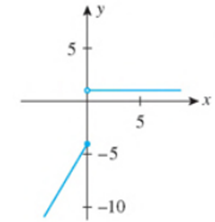Chapter 2.5, Problem 39E

Chapter
Section
Textbook Problem

In Exercises 39-44, determine the values of x, if any, at which each function is discontinuous. At each number where f is discontinuous, state the condition(s) for continuity that are violated.39.f ( x ) = { 2 x − 4 if  x ≤ 0 1 if  x > 0

To determine

To find: The value of x at which the function f(x)={2x4ifx01ifx>0 is discontinuous.

Explanation

Definition used:

The function will be continuous when the value of left hand limit and the value of right hand limit is equal to the value of the function at that point and value of that function should satisfy that point.

A function f is continuous at x=a when it follows the following three conditions,

(1) f(a) is defined.

(2) limxaf(x) exists.

(3) limxaf(x)=f(a).

Calculation:

The given function is f(x)={2x4ifx01ifx>0 (1)

The graph of the given function is shown below,

Figure (1)

Substitute 0 for x in equation (1) to find the left hand limit,

limx0f(x)=limx0(2x4)=2(0)4=04=4

Substitute 0 for x in equation (1) to find the right hand limit,

Still sussing out bartleby?

Check out a sample textbook solution.

See a sample solution

The Solution to Your Study Problems

Bartleby provides explanations to thousands of textbook problems written by our experts, many with advanced degrees!

Get Started

Find the general indefinite integral. (x2+1+1x2+1)dx

Single Variable Calculus: Early Transcendentals, Volume I

Evaluate the integral. 19xdx

Calculus (MindTap Course List)

In problems 37-44, perform the indicated operations and simplify. 43.

Mathematical Applications for the Management, Life, and Social Sciences